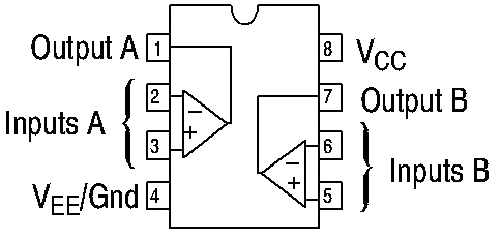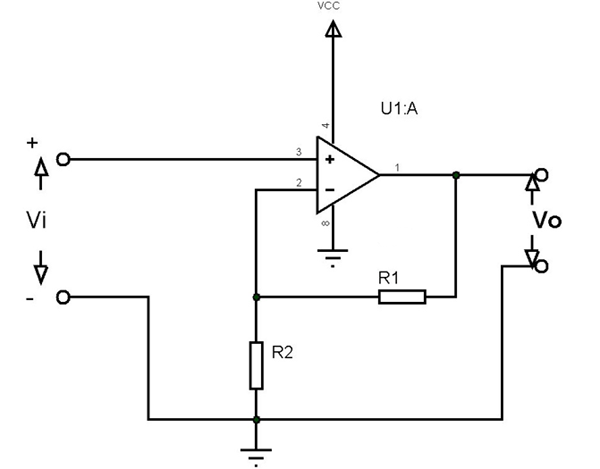# CA3240 Dual Operational Amplifier

[Click the image to enlarge it]

The CA3240 is a dual version of the popular CA3140 series integrated circuit operational amplifiers. CD3240 combines the advantages of MOS and bipolar transistors on the same monolithic chip. The gate-protected MOSFET input transistors provide high input impedance and a wide common-mode input voltage range (typically to 0.5V below the negative supply rail).

### CA3240 Pin Configuration

 Pin Number Pin Name Description 1 OUT (A) The output pin of the Op-amp A 2 Inverting Input (A) The Inverting input pin of the Op-Amp A 3 Non- Inverting Input (A) The Non - Inverting Input Pin of Amplifier A 4 Power (-Vs) Negative supply terminal 5 Reference The Non - Inverting Input Pin of Amplifier B 6 Output The Inverting input pin of the Op-Amp B 7 Power (+Vs) The output pin of the Op-amp B 8 +VS Positive supply terminal

### Features and Specifications of CA3240

• Number of Channels:2 Channel
• Can work from a single power supply
• GBP - Gain Bandwidth Product: 4.5 MHz
• Supply Voltage - Max: 36 V
• Supply Voltage - Min: 4 V
• Maximum Dual Supply Voltage: +/- 18 V
• Minimum Dual Supply Voltage: +/- 2 V
• Operating Supply Current: 8 mA
• Output Current per Channel: 40 mA
• SR - Slew Rate: 9 V/us
• CMRR - Common Mode Rejection Ratio: 70 dB
• Vos - Input Offset Voltage:15 mV

Note:  Complete Technical Details can be found at the CA3240 datasheet given at the end of this page.

Similar Op-Amp. ICs

LM158, LM158A, LM358, LM358A, LM2904, LM2904Q, LM4558, LM747

### Where to Use CA3240 Op Amp

CA3240 IC can be used to realize general-purpose op-amp operation circuits like comparator, differential amplification or mathematical operations. Moreover, the device specifically designed for applications like transducer amplifiers, dc amplification blocks to be implemented more easily in single-supply-voltage systems. For example, these devices can be operated directly from the standard 5V supply used in digital electronics without additional -5V supply.

In addition with two of those op-amps on board, the device can perform two different functions at a time that comes in handy in applications. The device is popular among hobbyists and engineers for being low cost and good performance.

### How to Use CA3240 Op Amp IC

As mentioned above CA3240 is a dual op-amp IC. The internal connection diagram of CA3240 is shown below.Now let us take a single op-amp from the two and construct a simple application circuit as shown below.In the above circuit, the op-amp acts as a Non-Inverting Amplifier (Since the input is given to the Non-inverting terminal of the op-amp) and output is provided as Vo. Also, the device is powered from a single voltage source of VCC.

We have the equation for the output Vo =Input voltage * Gain = Vi * A = Vi * (1+R1/R2)

For example:

Say R1 = 100, R2 = 10 and Input voltage Vi = 20mV

We will have Vo = 20m*(1+10)=220mV

With that, we have realized the amplifier circuit of the op-amp and in a similar way, we use the device to design all other application circuits of an op-amp.

### Applications

• Ground Referenced Single Amplifiers in Automobile and
• Portable Instrumentation
• Sample and Hold Amplifiers
• Long Duration Timers/Multivibrators (Microseconds Minutes-Hours)
• Photocurrent Instrumentation
• Intrusion Alarm System
• Comparators
• Function Generators
• Instrumentation  Amplifiers
Component Datasheet